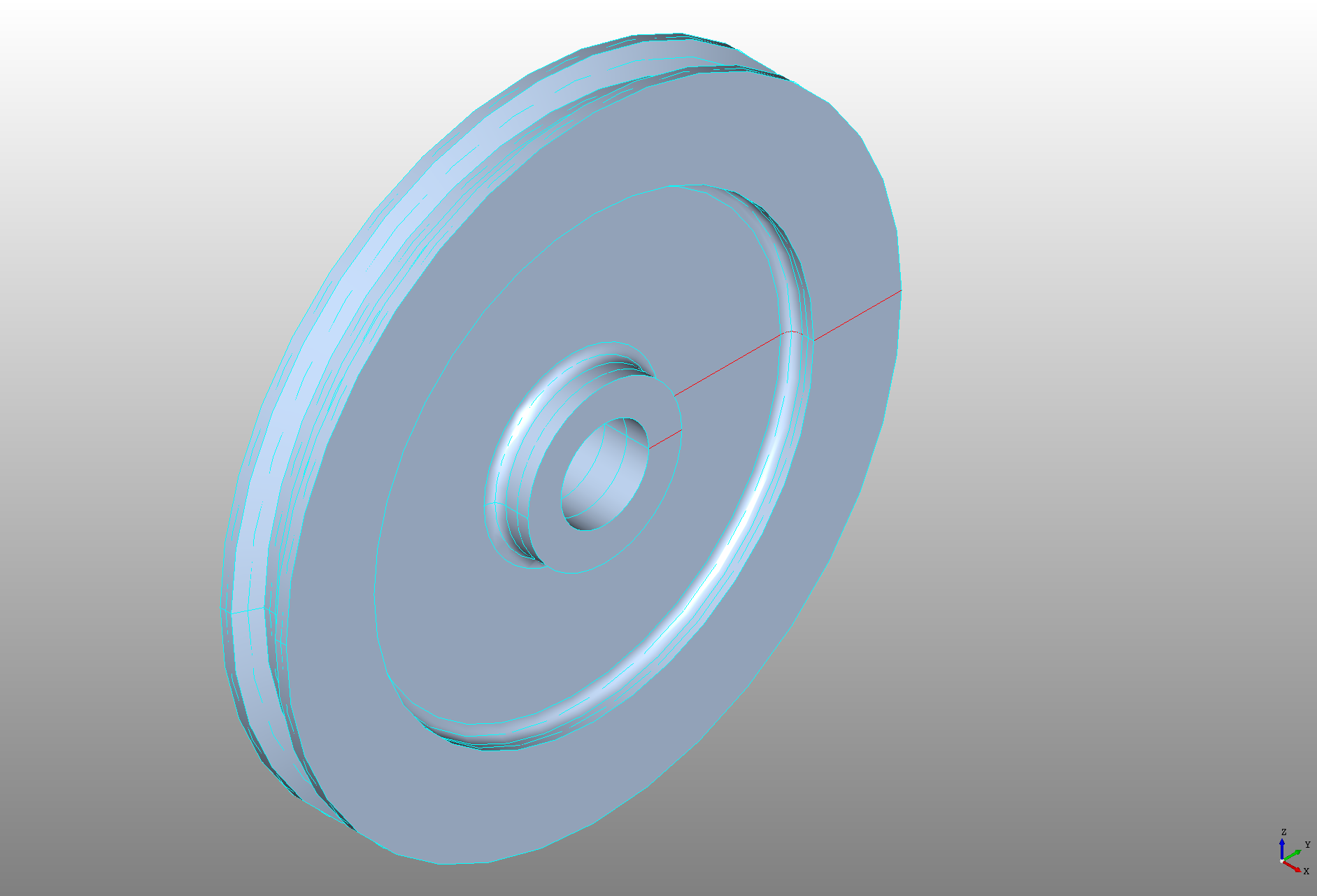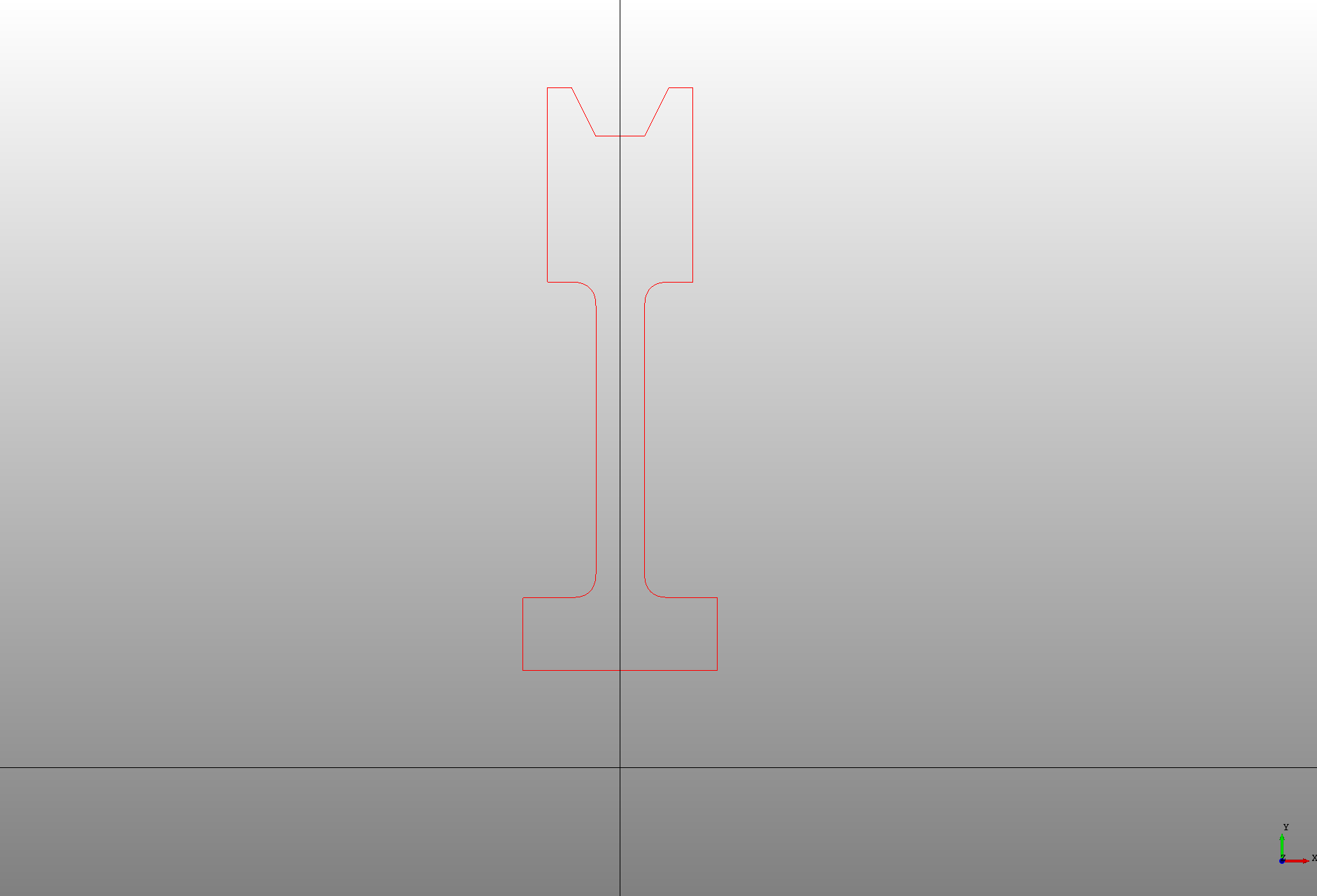## Revolve

The `Revol` declaration is used to revolve a profile around an axis. The axis of revolution is defined by the `position`, `direction`, and `rotation` of the `Revol`. By default it revolves a full rotation, this can be limited by setting the `angle` attribute in radians.

Like the `Prism` a wire will create a surface and a face or closed wire will create a solid shape.

``````# Created in DeclaraCAD

enamldef Profile(Wire):
attr r = 1 # Shaft radius
attr r2 = 7 # Total radius
attr r3 = 5 # Groove radius
attr w1 = 2 # Width at base
attr w2 = 1.5 # Wdth at top
attr f1 = 0.25 # Fillet radius
attr t = 0.25 # Thickness
attr t2 = 0.5 # Groove thickness
attr d1 = 0.5 # Groove depth
Wire: wire:
Polyline: p1:
points = [
(0, r),
(w1/2, r),
(w1/2, r + t*3),
(t + f1, r + t*3),
]
Bezier: b1:
attr p = p1.points[-1]
points = [
p, p + (-f1, 0), p + (-f1, f1)
]
Segment: s1:
attr p = b1.points[-1]
attr d = r3 - t*3 - r - 2*f1
points = [p, p + (0, d)]
Bezier: b2:
attr p = s1.points[-1]
points = [
p, p + (0, f1), p + (f1, f1)
]
Polyline: p2:
attr p = b2.points[-1]
points = [
p,
(w2/2, r3),
(w2/2, r2),
(w2/2-t, r2), # TODO: Determine based on angle
(t2/2, r2-d1),
(0, r2-d1),
]
Transform:
operations = [Mirror(y=1)]
shape = wire

enamldef Assembly(Part):
Profile: profile:
color = 'red'
Revol:
color = 'lightsteelblue'
direction = (1, 0, 0)
shape = profile
``````

The direction is set to `(1, 0, 0)` so it revolves around the the X-axis at the origin.This is the profile that was created in the XY plane.